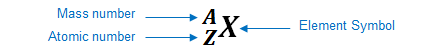# Problem: How many protons, electrons, and neutrons are present in an atom of copper whose atomic number is 29 and whose mass number is 64?

###### FREE Expert Solution

An element can be represented as:You can determine the identity of the element using its atomic number:

Atomic number (Z):

represents the identity of the element
reveals the number of protons
in a neutral element:

# of protons = # of electrons

Mass number (A)

reveals the number of protons and neutrons

mass number = # of protons + # of neutrons

95% (432 ratings)###### Problem Details

How many protons, electrons, and neutrons are present in an atom of copper whose atomic number is 29 and whose mass number is 64?